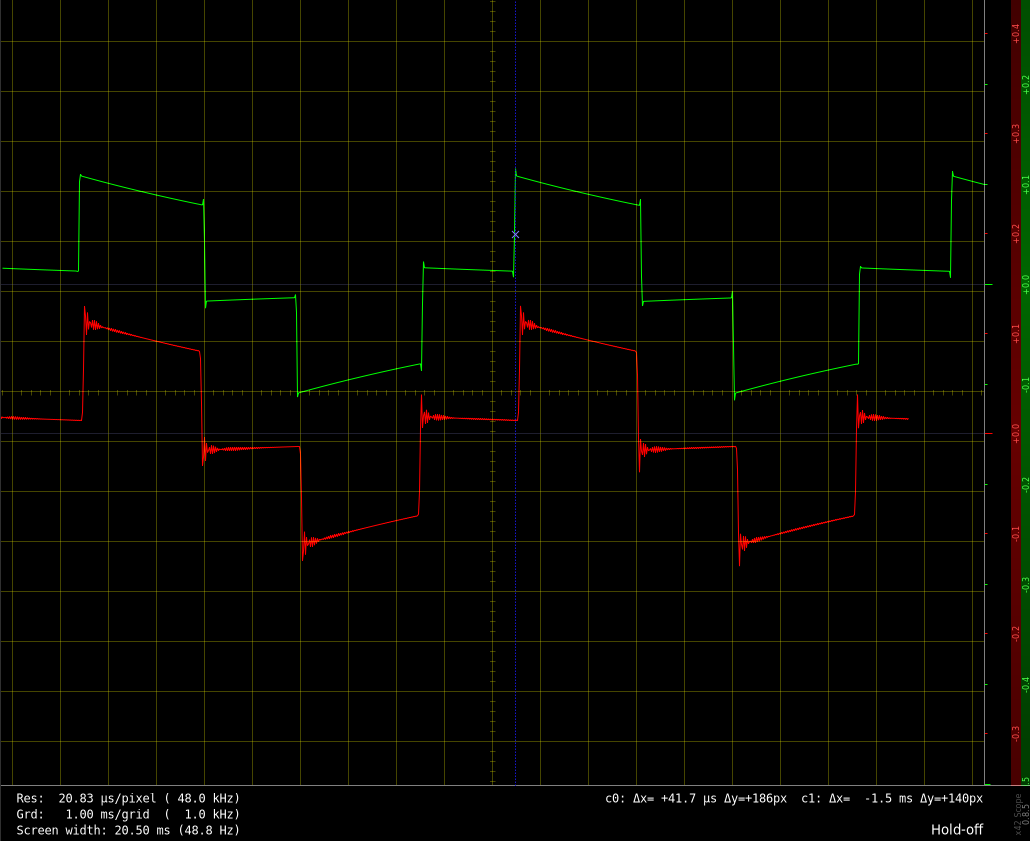# Effects

## Disto

Rough approximation `cutoff = 440*2**((disto_tone*1.45-69)/12)`

Consider using the same formula as reverb below.

## Reverb

• `reverb_tone` 0-100 is the cutoff of a low pass filter

The cutoff is analyzed by playing a sound in 2 instances, one with reverb_tone=100 and varying the tone of the other. Compute Y(z)/X(z) of the two spectrums and identify the -3dB point of the magnitude.

The result is approximately `21+(0.01*reverb_tone)*108` expressed in midi pitch units.

## Automatic pannner

This is the automatic panner.

``````/**
Auto-panner

Frequency: same as `apan_freq`
Phase offset: same as `apan_phase`

Other:
- `apan_waveform`: LFO wave number (cf. `lfoN_wave`)
- `apan_depth`:
0 to disable, >0 to enable (reference)
jpc: in this implementation, depth is allowed 0-100% granularity
to make it more meaningful

Note:
Reference uses a linear pan function.
Below, logarithmic is provided but it's commented.
*/

import("stdfaust.lib");

f = hslider(" Frequency [unit:Hz]", 5.0, 0.01, 5.0, 0.01);
o = hslider(" Phase offset [unit:deg]", 180.0, 0.0, 360.0, 1.0) : *(1.0/360.0);
d = hslider(" Depth [unit:%]", 50, 0, 100, 1) : *(0.01);

autopan = *(panL(lfo01)), *(panR(lfo01))
with {
// any chosen waveform with output in domain [0:1]
calcLfo = triangle;

// output of the overall LFO, which modulates left and right exactly opposite
// it's the difference of 2 sub-LFO with a phase offset between them
lfoDD = d*(calcLfo(phase1)-calcLfo(phase2)); // outputs [-d:+d]
lfo01 = 0.5*(lfoDD+1.0); // outputs [0:1]

// running phase of 2 synced LFO with fixed offset
phase1 = os.lf_sawpos(f);
phase2 = wrap(phase1+o);

// ordinary stereo pan function (+3dB)
//panL(x) = cos(x*(ma.PI/2.0))*sqrt(2.0);
//panR(x) = sin(x*(ma.PI/2.0))*sqrt(2.0);

// linear stereo pan function
panL(x) = 2.*(1.-x);
panR(x) = 2.*(x);

triangle(p) = 1.-abs(2.*p-1.);
wrap(x) = x-int(x);
};

/* Test */
process = autopan;
// process = os.osci(110.0) : *(g) <: (_, _) : autopan with {
//   g = hslider(" Gain adjust", 0.4, 0.1, 1.0, 0.01);
// };
``````

## Bit reduction

Matched `bitred` effect with 0-100 control range. Implementation below is naive, it needs antialiasing.

Note: reference seems using a transition function to reduce aliasing between jumps from a quantization step to another.

PolyBLEP: http://www.martin-finke.de/blog/articles/audio-plugins-018-polyblep-oscillator/

``````import("stdfaust.lib");

amount = hslider("Amount", 90, 0, 100, 1);
oversampling = 1;//fconstant(int gOversampling, <math.h>);

bitred = *(steps) : roundToInt : /(steps) : hpf1p(20.0/oversampling) with {
roundToInt(x) = ba.if(x<0, ma.neg(a), a) with { a = int(abs(x)); };
steps = 5.+(1.-amount*0.01)*512;
};

hpf1p(f) = fi.iir((0.5*(1.+p),-0.5*(1+p)),(0.-p)) with {
p = exp(-2.*ma.PI*f/ma.SR);
};

/* Test */
process = os.osci(110.0/oversampling) : *(0.15) : bitred <: (_, _);
``````

Figure: reference effect 99% on 110Hz sine wave (green), implementation with hiir oversampling 2x (red). The tiny peak between steps suggests a use of transition function (like polyBLEP)## Decimation

Matched by exponential fit on the target sampling frequency, in 0-100 range.

Same remarks apply as for `bitred` regarding polyBLEP, it's the same.

``````import("stdfaust.lib");

amount = hslider("Amount", 99, 0, 100, 1);

decim(x) = y : hpf1p(20.0) letrec {
'y = ba.if(p+(f/ma.SR)>1.0, x, y);
'p = wrap(p+(f/ma.SR)); // the position 0-1
}
with {
// exponential curve fit
a=5.729950e+04; b=-6.776081e-02; c=180;
f=a*exp(amount*b)+c;
};

hpf1p(f) = fi.iir((0.5*(1.+p),-0.5*(1+p)),(0.-p)) with {
p = exp(-2.*ma.PI*f/ma.SR);
};

wrap(x) = x-int(x);

/* Test */
process = os.osci(110.0) : *(0.15) : decim <: (_, _);
``````

## Phaser

Not complete, only frequency range was analyzed. Filter has not been matched, feedback not yet analyzed. See notes in comments.

``````/*
Dual-notch Phaser, with linear cutoff control by LFO

Note:
`phaser_stages`: number of stages. Cascade this function as many times as
needed to produce the effect.
`phaser_waveform`: wave number (same as LFO)
`phaser_freq`: frequency of LFO which modulates the notches
`phaser_depth`: manipulates the 2 ranges of cutoff for the notches
`phaser_feedback`: TODO, implemented in model below but not measured
`phaser_phase[_oncc]`: TODO, assumed to be the phase 0-1 of LFO?
*/

import("stdfaust.lib");

phaser = (+:notch1:notch2) ~ *(feedback) with {
depth = hslider(" Depth [unit:%]", 50, 0, 100, 1);
lfoFreq = hslider(" LFO frequency [unit:Hz]", 1.0, 0.0, 10.0, 0.01);

feedback = 0.0; // TODO determine `phaser_feedback` in the domain 0-100

lfo = os.lf_triangle(lfoFreq); // set any LFO waveform `phaser_waveform`

cutoffDepth1 = 31*depth;
cutoffMax1 = 3100.0;
cutoffCenter1 = 1600.0;

cutoffDepth2 = 155*depth;
cutoffMax2 = 13400.0;
cutoffCenter2 = 8300.0;

widthAdjust = 0.5; // arbitrary pick, not checked